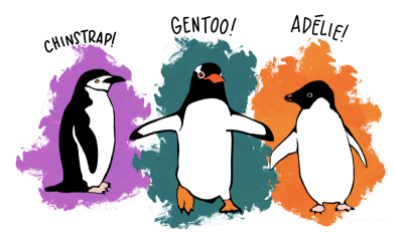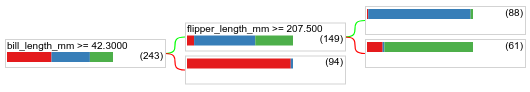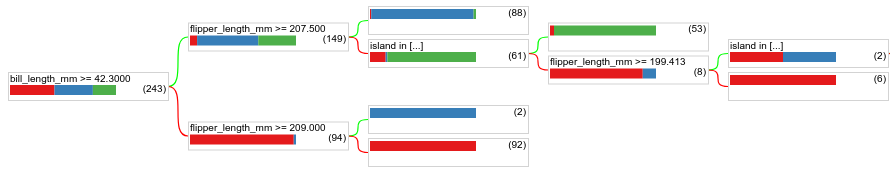# Creating a decision tree

In this unit, you'll use the TF-DF (TensorFlow Decision Forest) library train, tune, and interpret a decision tree.

## Preliminaries

Before studying the dataset, do the following:

1. Create a new Colab notebook.
2. Install the TensorFlow Decision Forests library by placing the following line of code in your new Colab notebook:
!pip install tensorflow_decision_forests

3. Import the following libraries:
import numpy as np
import pandas as pd
import tensorflow_decision_forests as tfdf


## The Palmer Penguins dataset

This Colab uses the Palmer Penguins dataset, which contains size measurements for three penguin species:

• Chinstrap
• Gentoo

This is a classification problem—the goal is to predict the species of penguin based on data in the Palmer's Penguins dataset.

Let’s meet the penguins.Figure 16. Three different penguin species. Image by @allisonhorst

The following code calls a pandas function to load the Palmer Penguins dataset into memory:

path = "https://storage.googleapis.com/download.tensorflow.org/data/palmer_penguins/penguins.csv"

# Display the first 3 examples.


The following table formats the first 3 examples in the Palmer Penguins dataset:

Table 3. The first 3 examples in Palmer Penguins

species island bill_length_mm bill_depth_mm flipper_length_mm body_mass_g sex year
0 Adelie Torgersen 39.1 18.7 181.0 3750.0 male 2007
1 Adelie Torgersen 39.5 17.4 186.0 3800.0 female 2007
2 Adelie Torgersen 40.3 18.0 195.0 3250.0 female 2007

The full dataset contains a mix of numerical (for example, bill_depth_mm), categorical (for example, island), and missing features. Unlike neural networks, decision forests support all these feature types natively, so you don't have to do one-hot encoding, normalization, or extra is_present feature.

To simplify interpretability, the following code cell manually converts the penguin species into integer labels:

label = "species"

classes = list(pandas_dataset[label].unique())
print(f"Label classes: {classes}")
# >> Label classes: ['Adelie', 'Gentoo', 'Chinstrap']

pandas_dataset[label] = pandas_dataset[label].map(classes.index)


The following code cell splits the dataset into a training set and testing set:

np.random.seed(1)
# Use the ~10% of the examples as the testing set
# and the remaining ~90% of the examples as the training set.
test_indices = np.random.rand(len(pandas_dataset)) < 0.1
pandas_train_dataset = pandas_dataset[~test_indices]
pandas_test_dataset = pandas_dataset[test_indices]

print("Training examples: ", len(pandas_train_dataset))
# >> Training examples: 309

print("Testing examples: ", len(pandas_test_dataset))
# >> Testing examples: 35


## Training a model with default hyperparameters

We can train our first CART (Classification and Regression Trees) model without specifying any hyperparameters. That's because the tfdf.keras.CartModel function provides good default hyperparameter values. You will learn more about how this type of model works later in the course.

tf_train_dataset = tfdf.keras.pd_dataframe_to_tf_dataset(pandas_train_dataset, label=label)
model = tfdf.keras.CartModel()
model.fit(tf_train_dataset)


The preceding call to tfdf.keras.CartModel did not specify columns to use as input features. Therefore, every column in the training set is used. The call also did not specify the semantics (for example, numerical, categorical, text) of the input features. Therefore, tfdf.keras.CartModel will automatically infer the semantics.

Call tfdf.model_plotter.plot_model_in_colab to display the resulting decision tree:

tfdf.model_plotter.plot_model_in_colab(model, max_depth=10)


In Colab, you can use the mouse to display details about specific elements.Figure 17. Visualization of a decision tree in Colab.

Colab shows that the root condition evaluated 277 examples. However, you might remember that the training dataset contained 309 examples. The remaining 32 examples were used for validation.

The first condition tests the value of bill_depth_mm. Tables 4 and 5 show the likelihood of different species depending on the outcome of the first condition.

Table 4. Likelihood of different species if bill_depth_mm ≥ 16.35

Species Likelihood
Chinstrap (green) 33%
Gentoo (blue) 4%

Table 5. Likelihood of different species if bill_depth_mm < 16.35

Species Likelihood
Chinstrap (green) 1%
Gentoo (blue) 97%

bill_depth_mm is a numerical feature. Therefore, the value 16.35 was found using the exact splitting for binary classification with numerical features algorithm.

If bill_depth_mm < 16.5 is True, further testing whether the body_mass_g ≥ 4175 can perfectly separate between 86 Gentoos and 5 Gentoos+Adelie.

The following code provides the training and test accuracy of the model:

model.compile("accuracy")
print("Train evaluation: ", model.evaluate(tf_train_dataset, return_dict=True))
# >> Train evaluation:  {'loss': 0.0, 'accuracy': 0.96116}

tf_test_dataset = tfdf.keras.pd_dataframe_to_tf_dataset(pandas_test_dataset, label=label)
print("Test evaluation: ", model.evaluate(tf_test_dataset, return_dict=True))
# >> Test evaluation:  {'loss': 0.0, 'accuracy': 0.97142}


It is rare, but possible, that the test accuracy is higher than the training accuracy. In that case, the test set possibly differs from the training set. However, this is not the case here as the train/test was split randomly. A more likely explanation is that the test dataset is very small (only 35 examples), so the accuracy estimation is noisy.

To train a better model, optimize the hyperparameters with the Keras tuner.

Important note: Don't tune hyperparameters on the test dataset. Instead, tune hyperparameters on a separate validation dataset (on large datasets) or using cross-validation (on small datasets). For simplicity, this course uses the test dataset for tuning.

The following code optimizes two parameters:

• the minimum number of examples in a condition node (min_examples)
• the ratio of the training dataset used for pruning validation (validation_ratio)

Since we don't know the best values for these parameters, we provide various possibilities for the tuner to try. We've picked four possible values for min_examples and three for validation_ratio. Increasing the number of candidate hyperparameter values increases the chance of training a better model, but it also increases the training time.

!pip install keras-tuner

import keras_tuner as kt

def build_model(hp):
model = tfdf.keras.CartModel(
min_examples=hp.Choice("min_examples",
# Try four possible values for "min_examples" hyperparameter.
# min_examples=10 would limit the growth of the decision tree,
# while min_examples=1 would lead to deeper decision trees.
[1, 2, 5, 10]),
validation_ratio=hp.Choice("validation_ratio",
# Three possible values for the "validation_ratio" hyperparameter.
[0.0, 0.05, 0.10]),
)
model.compile("accuracy")
return model

tuner = kt.RandomSearch(
build_model,
objective="val_accuracy",
max_trials=10,
directory="/tmp/tuner",
project_name="tune_cart")

tuner.search(x=tf_train_dataset, validation_data=tf_test_dataset)
best_model = tuner.get_best_models()

print("Best hyperparameters: ", tuner.get_best_hyperparameters().values)
# >> Best hyperparameters:  {'min_examples': 2, 'validation_ratio': 0.0}


The candidate values of the hyperparameter (for example, [1,2,5,10] for min_examples) depend on your understanding of the dataset and the amount of computing resources available.

The following code retrains and evaluates the model using those optimized hyperparameters:

model = tfdf.keras.CartModel(min_examples=2, validation_ratio=0.0)
model.fit(tf_train_dataset)

model.compile("accuracy")
print("Test evaluation: ", model.evaluate(tf_test_dataset, return_dict=True))
# >> Test evaluation:  {'loss': 0.0, 'accuracy': 1.0}


The accuracy of 1.0 means our model perfectly explains our test dataset in this toy example.

The following code plots the new decision tree:

tfdf.model_plotter.plot_model_in_colab(model, max_depth=10)Figure 18. A decision tree with six levels of nodes.

As expected by the new hyperparameter values, this decision tree is deeper than before because:

• The minimum number of examples was reduced (from 5 to 2).
• Validation pruning was disabled (validation_ratio=0.0) leading to more available training examples and no pruning.

## Usage and limitation

As mentioned earlier, a single decision tree often has lower quality than modern machine learning methods like random forests, gradient boosted trees, and neural networks. However, decision trees are still useful in the following cases:

• As a simple and inexpensive baseline to evaluate more complex approaches.
• When there is a tradeoff between the model quality and interpretability.
• As a proxy for the interpretation of the decision forests model, which the course will explore later on.
[{ "type": "thumb-down", "id": "missingTheInformationINeed", "label":"Missing the information I need" },{ "type": "thumb-down", "id": "tooComplicatedTooManySteps", "label":"Too complicated / too many steps" },{ "type": "thumb-down", "id": "outOfDate", "label":"Out of date" },{ "type": "thumb-down", "id": "samplesCodeIssue", "label":"Samples / code issue" },{ "type": "thumb-down", "id": "otherDown", "label":"Other" }]
[{ "type": "thumb-up", "id": "easyToUnderstand", "label":"Easy to understand" },{ "type": "thumb-up", "id": "solvedMyProblem", "label":"Solved my problem" },{ "type": "thumb-up", "id": "otherUp", "label":"Other" }]